ICSE Class 9 Indices Solution New Pattern

## ICSE Class 9 Indices Solution New Pattern By Clarify Knowledge

ICSE Class 9 Indices Solution New Pattern 2022

## Chapter 7 - Indices (Exponents) Exercise Ex. 7(A)

Question 1

Evaluate:

(i)(ii)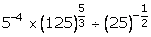(iii)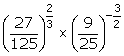(iv)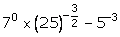(v)Solution 1

(i)

(ii)

(iii)

(iv)

(v)

Question 2

Simplify:

(i)(ii)(iii)(iv)Solution 2

(i)

(ii)

(iii)

(iv)

Question 3

Evaluate:

(i)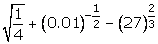(ii)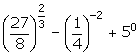Solution 3

(i)

(ii)

Question 4

Simplify each of the following and express with positive index:

(i)(ii)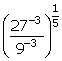(iii)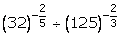(iv)Solution 4

(i)

(ii)

(iii)

(iv)

Question 5

If 2160 = 2a. 3b. 5c, find a, b and c. Hence calculate the value of 3a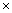2-b5-c.Solution 5

Question 6

If 1960 = 2a. 5b. 7c, calculate the value of 2-a. 7b. 5-c.Solution 6

Question 7

Simplify:

(i)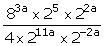(ii)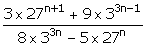Solution 7

(i)

(ii)

Question 8

Show that:

Solution 8

Question 9

If a = xm + n. yl; b = xn + lym and c = xl + m. yn,

Prove that: am - n. bn - l. cl - m = 1Solution 9

Question 10

Simplify:

(i)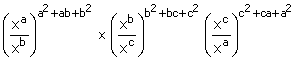(ii)Solution 10

(i)

(ii)

## Chapter 7 - Indices (Exponents) Exercise Ex. 7(B)

Question 1

Solve for x:

(i) 22x+1 = 8

(ii) 25x-1 = 4 23x + 1

(iii) 34x + 1 = (27)x + 1

(iv) (49)x + 4 = 72 (343)x + 1Solution 1

(i)

(ii)

(iii)

(iv)

Question 2

Find x, if:

(i)

(ii)

(iii)

(iv) Solution 2

(i)

(ii)

(iii)

(iv)

Question 3

Solve:

(i) 4x - 2 - 2x + 1 = 0

(ii) Solution 3

(i)

(ii)

Question 4

Solve :

(i) 8 22x + 4 2x+1 = 1 + 2x

(ii)22x + 2x+2 - 4 23 = 0

(iii) Solution 4

(i)

(ii)

(iii)

Question 5

Find the values of m and n if:

Solution 5

Question 6

Solution 6

Question 7

Prove that:

(i) =1

(ii) Solution 7

(i)

(ii)

Question 8

If ax = b, by = c and cz = a, prove that: xyz = 1.Solution 8

Question 9

If ax = by = cz and b2 = ac, prove that :Solution 9

Question 10

If 5-P = 4-q = 20r, show that:

Solution 10

Question 11

If m ≠ n and (m + n)-1 (m-1 + n-1) = mxny, show that:

x + y + 2 = 0Solution 11

Question 12

If 5x + 1 = 25x - 2, find the value of

3x - 3 × 23 - xSolution 12

Question 13

If 4x + 3 = 112 + 8 × 4x, find the value of (18x)3x.Solution 13

Question 14 (i)

Solve for x:

4x-1 × (0.5)3 - 2x = Solution 14 (i)

Question 14 (ii)

Solve for x:

(a3x + 5)2. (ax)4 = a8x + 12Solution 14 (ii)

Question 14 (iii)

Solve for x:

Solution 14 (iii)

Question 14 (iv)

Solve for x:

23x + 3 = 23x + 1 + 48Solution 14 (iv)

Question 14 (v)

Solve for x:

3(2x + 1) - 2x + 2 + 5 = 0Solution 14 (v)

Question 14 (vi)

9x+2 = 720 + 9xSolution 14 (vi)

9x+2 = 720 + 9x

⇒ 9x+2 - 9x = 720

⇒ 9x (92 - 1) = 720

⇒ 9x (81 - 1) = 720

⇒ 9x (80) = 720

⇒ 9x = 9

⇒ 9x = 91

⇒ x = 1

## Chapter 7 - Indices (Exponents) Exercise Ex. 7(C)

Question 1

Solution 1

Question 2

Solution 2

Question 3

Solve: 3x-1× 52y-3 = 225.Solution 3

Question 4

Solution 4

Question 5

If 3x+1 = 9x-3, find the value of 21+x.Solution 5

Question 6

Solution 6

Question 7

Solution 7

Question 8

Solution 8

Question 9

Solution 9

Question 10

Solve: 3(2x + 1) - 2x+2 + 5 = 0.Solution 10

Question 11

If (am)n = am .an, find the value of:

m(n - 1) - (n - 1)Solution 11

Question 12

Solution 12

Question 13

Solution 13

Question 14

Solution 14

Question 15 (i)

Solution 15 (i)

Question 15 (ii)

Solution 15 (ii)

Question 16

Solution 16

error: Content is protected !!